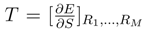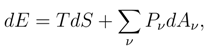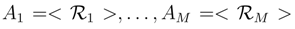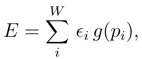Next Article in Journal / Special Issue
Generalised Exponential Families and Associated Entropy Functions
Previous Article in Journal
Entropy Generation and Human Aging: Lifespan Entropy and Effect of Physical Activity Level
Previous Article in Special Issue
Estimating the Entropy of Binary Time Series: Methodology, Some Theory and a Simulation Study

Article Menu
Metrics 0

## Export Article

Entropy 2008, 10(2), 124-130; https://doi.org/10.3390/entropy-e10020124

Article
Incremental Entropy Relation as an Alternative to MaxEnt
1
Exact Sciences Faculty, National University La Plata (UNLP), IFLP-CCT-CONICET, Argentina
2
CREG-UNLP and Conicet, Argentina; Department of Physics, University of Pretoria, South Africa; Instituto Carlos I de Fisica Teorica y Computacional, Universidad de Granada, Spain
3
CBPF, Rio de Janeiro, Brazil
4
Departament de Fisica and IFISC, Universitat de les Illes Balears, 07122 Palma de Mallorca, Spain
*
Author to whom correspondence should be addressed.
Received: 5 March 2008; in revised form: 14 June 2008 / Accepted: 22 June 2008 / Published: 24 June 2008

## Abstract

:
We show that, to generate the statistical operator appropriate for a given system, and as an alternative to Jaynes’ MaxEnt approach, that refers to the entropy S, one can use instead the increments δS in S. To such an effect, one uses the macroscopic thermodynamic relation that links δS to changes in i) the internal energy E and ii) the remaining M relevant extensive quantities Ai, i = 1, . . . , M, that characterize the context one is working with.
Keywords:
MaxEnt; probability density function; Thermodynamics’ relations

## 1. Introduction

Here we wish to address an issue belonging to the foundations of statistical mechanics (SM) by revisiting the role of the entropy S in Jaynes’ SM-formulation [1, 2], based upon the MaxEnt axiom: entropy is to be extremized (with suitable constraints). Of course, SM microscopically “explains” thermodynamics. The later can be axiomatized, as it is well-known, using four macroscopic postulates . Now, Jaynes’ axioms for SM and those of thermodynamics belong to different worlds altogether. The former speak of “observers’ ignorance”, a concept germane to the thermodynamics language, that refers to laboratory-parlance. Of course, there is nothing to object in this respect. However, one might ask whether it would be possible to look for a SM-“Jaynes’ counterpart” that speaks a similar language to that of thermodynamics. We shall address this issue below and try to provide answers. Our starting point is a brief re-visitation of thermodynamics’ axioms. Its four postulates are enumerated below and they are entirely equivalent to the celebrated three laws of thermodynamics :
• For every system there exists a quantity E, called the internal energy, such that a unique E−value is associated to each of its states. The difference between such values for two different states in a closed system is equal to the work required to bring the system, while adiabatically enclosed, from one state to the other.
• There exist particular states of a system, called the equilibrium ones, that are uniquely determined by E and a set of, say M, extensive (macroscopic) parameters Rν. The number and characteristics of the Rν depends on the nature of the system .
• For every system there exists a state function S(E, ∀Rν) that (i) always grows if internal constraints are removed and (ii) is a monotonously (growing) function of E. S remains constant in quasi-static adiabatic changes.
• S and the temperaturevanish for the state of minimum energy and are ≥ 0 for all other states.
From axiom 3 ones extracts, in particular, the following two statements, essential for our purposes
• Statement 3a) for every system there exists a state function S, a function of E and the Rν
S = S(E, R1,. . . , RM).
• Statement 3b) S is a monotonous (growing) function of E, so that one can interchange the roles of E and S in (1) and write
E = E(S, R1,. . . , RM),
Eq. (2) clearly indicates thatwith Pν generalized pressures and the temperature T defined as## 2. Our goal

We will show here, as our goal, that one can give Eq. (3) the status of an axiom of statistical mechanics! We introduce first a set of new extensive quantities Aν, appropriately related (see below) to the Rν and postulate for statistical mechanics that (Axiom (1), the incremental entropy postulate)a macroscopic statement whose microscopic import will become evident if we establish the relation between the Rν and the Aν (Axiom 2 below). This entails obviously that more is needed for the microscopic theory one is here building up. The minimum amount of microscopic information that we would have still to add to our axiomatics in order to get all the results of equilibrium statistical mechanics is precisely such relation. At this point we will merely conjecture that the following statements might suffice:
Axiom (2)
If there are W microscopic accessible states labelled by i, of microscopic probability pi, then
• (2-i)and
• (2-ii)
$E = E ( F )$
where $F$ stands for any set of additional quantities on which S and E may putatively also depend. Moreover,
• (2-iii)E and the external parameters are now to be regarded as expectation values of suitable operators, respectively the Hamiltonian H and the quantum operators corresponding to the macroscopic quantities Rν, to be here called $R ν$, so that Aν ≡ < $R ν$ >.
Thus the Aν, and also E (we realize at this stage), will depend on the eigenvalues of these operators and on the probability set. One may recognize now that Axiom (2) is just a form of Boltzmann’s “atomic” conjecture, pure and simple: macroscopic quantities are statistical averages evaluated using a microscopic probability distribution . In order to prove that the above two postulates indeed allow one to erect the mighty SM-edifice we show below that they are equivalent to Jaynes’ SM-axiomatics . A brief sketch reviewing MaxEnt follows, for the reader’s benefit.

#### 2.1. Information theory and the MaxEnt approach of Jaynes’

The main idea of information theory (IT) is to associate a degree of knowledge (or ignorance) I to any normalized probability distribution (PD) pi, (i = 1, . . . , W), determined by a functional of the {pi}. I is called an information measure [6,7,8]. Shannon, IT’s founder [7,8], proposed for I in 1948 the formk being an appropriate information unit (for instance, the bit). The quantum I−version replaces the probability distribution by the density operator ρ and the sum by the Trace operation. The main SMobjective thus gets translated into the issue of finding the PD (or the density operator) that best describes the system of interest. Jaynes appeals for this to his MaxEnt postulate, the only one needed in his formulation : MaxEnt axiom. Assume your prior knowledge about the system is given by the values of M expectation valuesThen, ρ is uniquely fixed by extremizing I(ρ) subject to ρ−normalization plus the constraints given by the M conditions constituting our assumed foreknowledgeThis leads, after a Lagrange-constrained extremizing process, to the introduction of M Lagrange multipliers λν, that one assimilates to the generalized pressures Pν . The truth, the whole truth, nothing but the truth . If the entropic measure that reflects our ignorance were not maximized, we would be inventing information that we do not possess.
In performing the variational process Jaynes discovers that, provided one sets k = kB in (8) (kB being Boltzmann’s constant) the information measure equals the entropic one. Thus, IS, the equilibrium thermodynamic entropy, with the caveat that our prior knowledgemust refer just to extensive quantities. Once ρ is at hand,yields complete microscopic information with respect to the system of interest.
The path to be followed should be clear now: we need to prove that the incremental entropy axiomatics, i.e., the set (5) - (7), is equivalent to MaxEnt.

## 3. The proof

We cover here only the classical instance. The quantal extension is of a straightforward character. We start with the generic differential change pipi + dpi, but constrained by Eq. (5). The differentials dpi must be of such character that (5) holds. Of course, S, Aj, and E will change with dpi and the concomitant changes are constrained by (5). We need not specify neither the explicit form of the information measure nor the way in which mean values are evaluated. In both cases, several possibilities have been advanced during the last 20 years . For a detailed discussion of this issue Ref.  is to be recommended. The ingredients of our scenario are
• an arbitrary, smooth function
IS({pi}),
such that S({pi}) is a concave function,
• M quantities Aν that represent mean values of extensive physical quantities $R ν$. These physical quantities $R ν$ take, for the micro-state i, the value $a i ν$ with probability pi,
• another arbitrary smooth, monotonic function g(pi) (g(0) = 0; g(1) = 1), that customarily (when the ordinary logarithmic Shannon entropy is used) is taken to be just g(pi) = pi.
We deal then with (we take A1E)where ϵi is the energy associated to the microstate i. The probability variations dpi in turn generate corresponding changes dS, dAν , and dE in, respectively, S, the Aν, and E.

#### 3.1. Part I

The essential point that we are introducing is to enforce obedience towith T the temperature and λν generalized pressures: λν = −Pν . We use now the expressions (11), (12), and (13) so as to cast (14) in terms of the probabilities, according to the change
pipi + dpi.
If we expand the resulting equation up to first order in the dpi it is immediately found that the following set of equations ensues [11,12] (remember that the Lagrange multipliers are identical to minus the generalized pressures Pν of Eq. (3)) :where primes denote pi−derivatives. Eq. (15) should yield one and just one pi−expression (one probability distribution), which it indeed does (see [11,12]). We do not need here, however, an explicit expression for this probability distribution, as will be immediately realized below.

#### 3.2. Part II

Alternatively, proceed à la MaxEnt. This requires extremizing the entropy S subject to the usual constraints in E, Aν, and normalization. The ensuing, Jaynes’ variational treatment is seen in [11,12], after appropriately dealing with delicate normalization-related issues [11,12], to yield the very set of Eqs. (15) as well! These equations arise then out of two clearly separate treatments: (I) our methodology, based on Eqs. (5) and (7), and (II), following the MaxEnt prescriptions. This entails that MaxEnt and our axiomatics co-imply each other, becoming thus equivalent ways of building up statistical mechanics.

## 4. Conclusions

We have seen that the set of equationsyields a probability distribution that coincides with the PD provided by either
• the MaxEnt’s, SM axiomatics of Jaynes’
• our two postulates: incremental entropy (5) and Boltzmann conjecture (7).
Let us repeat then, in our instance the postulates start with
• the macroscopic thermodynamic relation dE = TdS + ∑ν PνdAν,, adding to it
• Boltzmann’s conjecture of an underlying microscopic scenario ruled by microstate probability distributions.
The two postulates combine then (i) a well-tested macroscopic result with (ii) a by now un uncontestable microscopic state of affairs (which was not the case in Boltzmann’s times!). Thus one may confidently assert that these two postulates are intuitively intelligible from a physical laboratory standpoint, as promised in the Introduction. We have found a Jaynes’s SM counterpart centered on a differential entropy.

## Acknowledgments

This work was partially supported by (i) the MEC grant FIS2005-02796 (Spain) and FEDER (EU), (ii) the grant FQM 2445, Junta de Andalucia (Spain), and (iii) PIP6036-CONICET (Argentine Agency).

## References

1. Jaynes, E.T. Papers on probability, statistics and statistical physics; Rosenkrantz, R.D., Ed.; Reidel: Dordrecht, The Netherlands, 1987. [Google Scholar]
2. Grandy, W.T.; Milonni, P.W. (Eds.) Physics and Probability. Essays in Honor of Edwin T. Jaynes; Cambridge University Press: Cambridge, NY, USA, 1993.
3. Desloge, E.A. Thermal physics; Holt, Rhinehart and Winston: New York, NY, USA, 1968. [Google Scholar]
4. The MaxEnt treatment assumes that these macrocopic parameters are the expectation values of appropiate operators.
5. Lindley, D. Boltzmann’s atom; The Free Press: New York, NY, USA, 2001. [Google Scholar]
6. Katz, A. Principles of Statistical Mechanics. The information Theory Approach; Freeman and Co.: San Francisco, CA, USA, 1967. [Google Scholar]
7. Shannon, C.E. A mathematical theory of communication. Bell System Technol. J. 1948, 27, 379–390. [Google Scholar] [CrossRef]
8. Cover, T.M.; Thomas, J.A. Elements of information theory; J. Wiley Sons: New York, NY, USA, 1991. [Google Scholar]
9. Gell-Mann, M.; Tsallis, C. (Eds.) Nonextensive Entropy: Interdisciplinary applications; Oxford University Press: Offord, UK, 2004.
10. Ferri, G.L.; Martinez, S.; Plastino, A. Equivalence of the four versions of Tsallis’s statistics. Journal of Statistical Mechanics 2005, 04009. [Google Scholar] [CrossRef]
11. Curado, E.; Plastino, A. Equivalence between maximum entropy principle and enforcing dU=TdS. Phys. Rev. E 2005, 72, 047103:1–047103:3. [Google Scholar]
12. Curado, E.; Plastino, A. Generating statistical distributions without maximizing the entropy. Physica A 2006, 365, 24–27. [Google Scholar]
Entropy EISSN 1099-4300 Published by MDPI AG, Basel, Switzerland RSS E-Mail Table of Contents Alert
Back to Top